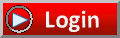Avatar Virtual Learning"Lowering College Education Costs for Everyone"CoursesTesting OutMy StuffFAQsAboutBiology (SMA-001)
The Biology examination covers material that is usually taught in a one-year college general biology course. The subject matter tested covers the broad field of the biological sciences, organized into three major areas: molecular and cellular biology, organismal biology and population biology.
Current status of course:
 Available

Calculus (SMA-002)
The Calculus examination covers skills and concepts that are usually taught in a one-semester college course in calculus. The content of each examination is approximately 60% limits and differential calculus and 40% integral calculus. Algebraic, trigonometric, exponential, logarithmic and general functions are included. The exam is primarily concerned with an intuitive understanding of calculus and experience with its methods and applications. Knowledge of preparatory mathematics is assumed, including algebra, geometry, trigonometry and analytic geometry.
Current status of course:
 In Development

Chemistry (SMA-003)
The Chemistry examination covers material that is usually taught in a one-year college course in general chemistry. Understanding of the structure and states of matter, reaction types, equations and stoichiometry, equilibrium, kinetics, thermodynamics, and descriptive and experimental chemistry is required, as is the ability to interpret and apply this material to new and unfamiliar problems. During this examination, an online scientific calculator function and a periodic table are available as part of the testing software.
Current status of course:
 In Development

College Algebra (SMA-004)
The College Algebra examination covers material that is usually taught in a one-semester college course in algebra. Nearly half of the test is made up of routine problems requiring basic algebraic skills; the remainder involves solving non-routine problems in which you must demonstrate your understanding of concepts. The test includes questions on basic algebraic operations; linear and quadratic equations, inequalities, and graphs; algebraic, exponential, and logarithmic functions; and miscellaneous other topics.
Current status of course:
 Available

College Mathematics (SMA-005)
The College Mathematics examination covers material generally taught in a college course for non-mathematics majors and majors in fields not requiring knowledge of advanced mathematics. Questions on the College Mathematics examination require you to demonstrate the ability to solve routine, straightforward problems and non-routine problems that require an understanding of concepts and the application of skills and concepts. In addition, you need to demonstrate understanding of topics like: sets, logic, real number systems, functions and graphs, probability and statistics, as well as topics in algebra and geometry.
Current status of course:
 In Development

Natural Sciences (SMA-006)
The Natural Sciences examination covers a wide range of topics frequently taught in introductory courses that survey both biological and physical sciences at the freshman or sophomore level. The primary objective of the exam is to give you the opportunity to demonstrate a level of knowledge and understanding expected of college students meeting a distribution or general education requirement in the natural sciences. The Natural Sciences exam is not intended for those specializing in science; it is intended to test the understanding of scientific concepts that an adult with a liberal arts education should have. It does not stress the retention of factual details; rather, it emphasizes the knowledge and application of the basic principles and concepts of science, the comprehension of scientific information, and the understanding of issues of science in contemporary society.
Current status of course:
 Available

Precalculus (SMA-007)
The Precalculus examination assesses the mastery of skills and concepts required for success in a first-semester calculus course. A large portion of the exam tests your understanding of functions and their specific properties: linear, quadratic, absolute value, square root, polynomial, rational, exponential, logarithmic, trigonometric, inverse trigonometric and piecewise-defined functions. Questions on the exam will present these types of functions symbolically, graphically, verbally or in tabular form. A solid understanding of these types of functions is at the core of all precalculus courses, and it is a prerequisite for enrolling in calculus and other college-level mathematics courses.
Current status of course:
 In Development